• 其中有两种简单又常用的插值算法用来实现图像缩放，分别是最近邻插值算法和双线性插值算法。 最近邻插值算法: 最近邻插值算法的思想十分简单 设原始图像src的高为h，宽为w，图像上像素值为(x,y)。 设目标图像dst...
图像缩放：
resize函数是在OpenCV中经常使用的函数，功能是将一副加载到Mat中的图像调整尺寸或者按照比例进行缩放。
其中有两种简单又常用的插值算法用来实现图像缩放，分别是最近邻插值算法和双线性插值算法。
最近邻插值算法:
最近邻插值算法的思想十分简单
设原始图像src的高为h，宽为w，图像上像素值为(x,y)。
设目标图像dst的高H，宽为W，图像上的像素值为(X,Y)。
那么根据比例缩放的思想有

xX=wW

x

X

=

w

W

$\frac{x}{X}=\frac{w}{W}$  同理，纵向上的像素对应比值为

yY=hH

y

Y

=

h

H

$\frac{y}{Y}=\frac{h}{H}$
那么在考虑目标图像dst上面的像素点(X,Y)对应到原始图像src上面像素点的位置为

(x,y)=(wWX,hHY)

(

x

,

y

)

=

(

w

W

X

,

h

H

Y

)

$(x,y)=(\frac{w}{W}X,\frac{h}{H}Y)$
这里要对

wWX

w

W

X

$\frac{w}{W}X$与

hHY

h

H

Y

$\frac{h}{H}Y$两个数值进行取整，在OpenCV源码当中，是用cvFloor函数取整，即舍去小数部分。
代码：
void InterNearestResize(const Mat &src, Mat &dst, Size &dsize, double fx = 0.0, double fy = 0.0)//原始图形以及缩放比例
{

Size ssize = src.size();//获取矩阵大小
CV_Assert(ssize.area() > 0);//保证矩阵的长宽都大于0

if (!dsize.area())//如果dsize为(0,0)
{
dsize = Size(saturate_cast<int>(src.cols*fx),//satureate_cast防止数据溢出
saturate_cast<int>(src.rows*fy));

CV_Assert(dsize.area());
}
else
{
fx = (double)dsize.width / src.cols;//Size中的宽高和mat中的行列是相反的
fy = (double)dsize.height / src.rows;
}

dst.create(Size(src.cols*fy,src.rows*fx), src.type());//将dst矩阵大小变为想要的尺寸

fx = 1.0 / fx;
fy = 1.0 / fy;
uchar *ps = src.data;
uchar *pd = dst.data;
int channels = src.channels(),x,y,out,in;

for (int row = 0; row < dst.rows; row++)
{
x = cvFloor(row * fx);
for (int col = 0; col < dst.cols; col++)
{
y = cvFloor(col * fy);
for (int c = 0; c < channels; c++)
{
out = (row * dst.cols + col) * channels + c;
in = (x * src.cols + y) * channels + c;
pd[out] = ps[in];
}

//dst.at<Vec3b>(row, col) = src.at<Vec3b>(x, y);
}
}
}
双线性插值算法:
如果原始图像src的大小是3×3，目标图像dst的大小是4×4，考虑dst中(1,1)点像素对应原始图像像素点的位置为(0.75,0.75)，如果使用最近邻算法来计算，原始图像的位置在浮点数取整后为坐标(0,0)。
上面这样粗暴的计算会丢失很多信息，考虑(0.75,0.75)这个信息，它表示在原始图像中的坐标位置，相比较取(0,0)点，(0.75,0.75)貌似更接近(1,1)点，那如果将最近邻算法中的取整方式改为cvRound（四舍五入）的方式取(1,1)点，同样会有丢的信息，即丢失了“0.25”部分的(0,0)点、(1,0)点和(0,1)点。
可以看到，dst图像上(X,Y)对应到src图像上的点，最好是根据计算出的浮点数坐标，按照百分比各取四周的像素点的部分。  如下图：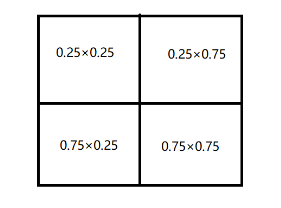双线性插值的原理相类似，这里不写双线性插值计算点坐标的方法，容易把思路带跑偏，直接就按照比率权重的思想考虑。
将

(wWX,hHY)

(

w

W

X

,

h

H

Y

)

$(\frac{w}{W}X,\frac{h}{H}Y)$写成

(x′+u,y′+v)

(

x

′

+

u

,

y

′

+

v

)

$(x'+u,y'+v)$的形式，表示将

x

x

$x$与y$y$$y$中的整数和小数分开表示

uv

u

v

$uv$分别代表小数部分。
这样，根据权重比率的思想得到计算公式

(X,Y)=(1−u)⋅(1−v)⋅(x,y)+(u−1)⋅v⋅(x,y+1)+u⋅(v−1)⋅(x+1,y)+(u⋅v)⋅(x,y)

(

X

,

Y

)

=

(

1

−

u

)

·

(

1

−

v

)

·

(

x

,

y

)

+

(

u

−

1

)

·

v

·

(

x

,

y

+

1

)

+

u

·

(

v

−

1

)

·

(

x

+

1

,

y

)

+

(

u

·

v

)

·

(

x

,

y

)

$(X,Y)=(1-u)·(1-v)·(x,y)+(u-1)·v·(x,y+1)+u·(v-1)·(x+1,y)+(u·v)·(x,y)$
在实际的代码编写中，会有两个问题，一个是图像会发生偏移，另一个是效率问题。
几何中心对齐：
由于计算的图像是离散坐标系，如果使用

(wWX,hHY)

(

w

W

X

,

h

H

Y

)

$(\frac{w}{W}X,\frac{h}{H}Y)$公式来计算，得到的(X,Y)值是错误的比率计算而来的，即(x+1,y)、(x,y+1)、(x+1,y+1)这三组点中，有可能有几个没有参与到比率运算当中，或者这个插值的比率直接是错误的。  例如，src图像大小是

a×a

a

×

a

$a×a$，dst图像的大小是

0.5a×0.5a

0.5

a

×

0.5

a

$0.5a×0.5a$。
根据原始公式计算

(wW,hH)

(

w

W

,

h

H

)

$(\frac{w}{W},\frac{h}{H})$得到

(2,2)

(

2

,

2

)

$(2,2)$（注意这不是表示点坐标，而是x和y对应的比率）
如果要计算dst点(0,0)对应的插值结果，由于

(2,2)

(

2

,

2

)

$(2,2)$是整数，没有小数，所以最后得到dst点在

(0,0)

(

0

,

0

)

$(0,0)$点的像素值就是src图像上在

(0,0)

(

0

,

0

)

$(0,0)$点的值。然而，我们想要的dst在

(0,0)

(

0

,

0

)

$(0,0)$上的结果是应该是有(0,0)(1,0)(0,1)(1,1)这四个点各自按照0.5×0.5的比率加权的结果。  所以我们要将dst上面的点，按照比率

(wW,hH)

(

w

W

,

h

H

)

$(\frac{w}{W},\frac{h}{H})$向右下方向平移0.5个单位。  公式如下：

(x,y)=(XwW+0.5(wW−1),YhH+0.5(hH−1))

(

x

,

y

)

=

(

X

w

W

+

0.5

(

w

W

−

1

)

,

Y

h

H

+

0.5

(

h

H

−

1

)

)

(x,y)=(X\frac{w}{W}+0.5(\frac{w}{W}-1),Y\frac{h}{H}+0.5(\frac{h}{H}-1))

运算优化：
由计算公式可以得知，在计算每一个dst图像中的像素值时会涉及到大量的浮点数运算，性能不佳。
可以考虑将浮点数变换成一个整数，即扩大一定的倍数，运算得到的结果再除以这个倍数。
举一个简单的例子，计算0.25×0.75，可以将0.25和0.75都乘上8，得到2×6=12，结果再除以

82

8

2

$8^2$，这样运算的结果与直接计算浮点数没有差别。
在程序中，没有办法取得一个标准的整数，使得两个相互运算的浮点数都变成类似“2”和”6“一样的标准整数，只能取一个适当的值来尽量的减少误差，在源码当中取值为

211

2

11

$2^{11}$=2048，即2的固定幂数，最后结果可以通过用位移来表示除以一个2整次幂数，计算速度会有很大的提高。
代码：
void Inter_Linear(const Mat &src, Mat &dst, Size &dsize ,double fx = 0.0, double fy = 0.0)//双线性插值
{
Size ssize = src.size();//获取矩阵大小
CV_Assert(ssize.area() > 0);//保证矩阵的长宽都大于0

if (!dsize.area())//如果dsize为(0,0)
{
dsize = Size(saturate_cast<int>(src.cols*fx),//satureate_cast防止数据溢出
saturate_cast<int>(src.rows*fy));

CV_Assert(dsize.area());
}
else
{
fx = (double)dsize.width / src.cols;//Size中的宽高和mat中的行列是相反的
fy = (double)dsize.height / src.rows;
}

dst.create(dsize, src.type());

double ifx = 1. / fx;
double ify = 1. / fy;

uchar* dp = dst.data;
uchar* sp = src.data;

int iWidthSrc = src.cols;//宽(列数）
int iHiehgtSrc = src.rows;//高(行数)
int channels = src.channels();
short cbufy;
short cbufx;

for (int row = 0; row < dst.rows; row++)
{
float fy = (float)((row + 0.5) * ify - 0.5);
int sy = cvFloor(fy);//整数部分
fy -= sy;//小数部分
sy = std::min(sy, iHiehgtSrc - 2);
sy = std::max(0, sy);

cbufy = cv::saturate_cast<short>((1.f - fy) * 2048);
cbufy = 2048 - cbufy;

for (int col = 0; col < dst.cols; col++)
{
float fx = (float)((col + 0.5) * ifx - 0.5);
int sx = cvFloor(fx);
fx -= sx;

if (sx < 0)
{
fx = 0, sx = 0;
}
if (sx >= iWidthSrc - 1)
{
fx = 0, sx = iWidthSrc - 2;
}

cbufx = cv::saturate_cast<short>((1.f - fx) * 2048);
cbufx = 2048 - cbufx;

for (int k = 0; k < src.channels(); ++k)
{
dp[(row * dst.cols + col) * channels + k] =
(
sp[ ( sy * src.cols + sx ) * channels + k] * cbufx * cbufy +
sp[((sy + 1) * src.cols + sx) * channels + k] * cbufx * cbufy +
sp[(sy * src.cols + (sx + 1)) * channels + k] * cbufx * cbufy +
sp[((sy + 1) * src.cols + (sx + 1)) * channels + k] * cbufx * cbufy
) >> 22;
}
}
}

}
参考博客
对应OpenCV源码部分为  opencv-2.4.9\modules\imgproc\src\imgwarp 部分
展开全文图像缩放
• 一. 最近邻插值法放大图像： ...最近邻插值算法原理 ↑ 二. 最近邻插值法算法流程： 插值法放大图像的第一步都是相同的，计算新图的坐标点像素值对应原图中哪个坐标点的像素值来填充，计算公式为： srcX = ...
一. 最近邻插值法放大图像：
最近邻插值法在放大图像时补充的像素是最近邻的像素的值。由于方法简单，所以处理速度很快，但是放大图像画质劣化明显，常常含有锯齿边缘。最近邻插值法算法原理 ↑

二. 最近邻插值法算法流程：
插值法放大图像的第一步都是相同的，计算新图的坐标点像素值对应原图中哪个坐标点的像素值来填充，计算公式为：
srcX = dstX * (srcWidth/dstWidth)
srcY = dstY * (srcHeight/dstHeight)
其中，src表示旧图，dst表示新图。新图的坐标（dstX,dstY）对应于旧图的坐标（srcX,srcY）。 srcWidth/dstWidth 和 srcHeight/dstHeight 分别表示宽和高的放缩比。
那么问题来了，通过这个公式算出来的 srcX,scrY （旧图坐标）有可能是小数，但是坐标点是不存在小数的，都是整数，得想办法把它转换成整数才行。
不同插值法的区别就体现在 srcX,scrY 是小数时，怎么变成整数去取原图像中的像素值。
最近邻插值法：看名字就很直白，四舍五入选取最接近的整数。这样的做法会导致像素的变化不连续，在新图中会产生锯齿。

三. 实验 python实现最近邻插值算法
from PIL import Image
import matplotlib.pyplot as plt
import numpy as np
import math

# 最近邻插值算法
# dstH为新图的高;dstW为新图的宽
def NN_interpolation(img,dstH,dstW):
scrH,scrW,_=img.shape
retimg=np.zeros((dstH,dstW,3),dtype=np.uint8)
for i in range(dstH-1):
for j in range(dstW-1):
scrx=round(i*(scrH/dstH))
scry=round(j*(scrW/dstW))
retimg[i,j]=img[scrx,scry]
return retimg

im_path='../paojie.jpg'
image=np.array(Image.open(im_path))

image1=NN_interpolation(image,image.shape*2,image.shape*2)
image1=Image.fromarray(image1.astype('uint8')).convert('RGB')
image1.save('out.png')

四. 实验结果：原图 ↑最近邻插值后图像 ↑
可以看到新图中产生了锯齿状的边缘。

五. 参考内容：
https://www.cnblogs.com/wojianxin/p/12515061.html
https://www.jianshu.com/p/4954643f7514
展开全文数字图像处理 图像放大 python实现
• 一、算法原理 提出此算法的背景是基于图片的缩放，在图片缩放的过程中，实质上就是将原图像像素矩阵像素值，填到目标图像像素矩阵中，目标图像像素矩阵可能比原图像像素矩阵大（图片放大），也可能小（图片缩小）。...
一、算法原理
提出此算法的背景是基于图片的缩放，在图片缩放的过程中，实质上就是将原图像像素矩阵像素值，填到目标图像像素矩阵中，目标图像像素矩阵可能比原图像像素矩阵大（图片放大），也可能小（图片缩小）。我们假设图片的宽(

W

i

d

t

h

Width

)和高(

H

e

i

g

h

t

Height

)是按同比例缩放的，那么

s

r

c

X

s

r

c

W

i

d

t

h

=

d

s

t

X

d

s

t

W

i

d

t

h

\frac{srcX}{srcWidth}= \frac{dstX}{dstWidth}

s

r

c

Y

s

r

c

H

e

i

g

h

t

=

d

s

t

Y

d

s

t

H

e

i

g

h

t

\frac{srcY}{srcHeight}=\frac{dstY}{dstHeight}

也就是，给定一个目标图片矩阵在(

d

s

t

X

dstX

,

d

s

t

Y

dstY

)处的坐标，计算出对应缩放前原图像的某点坐标(

s

r

c

X

srcX

,

s

r

c

Y

srcY

)，将后者的像素RGB值填入前者。但在计算中常常遇到算出的(

s

r

c

X

srcX

,

s

r

c

Y

srcY

)为浮点型的情况，如图1。而在像素坐标中，所有的坐标都应该为整型，因此本文的这两个算法就是为了解决浮点型原图像坐标的处理问题。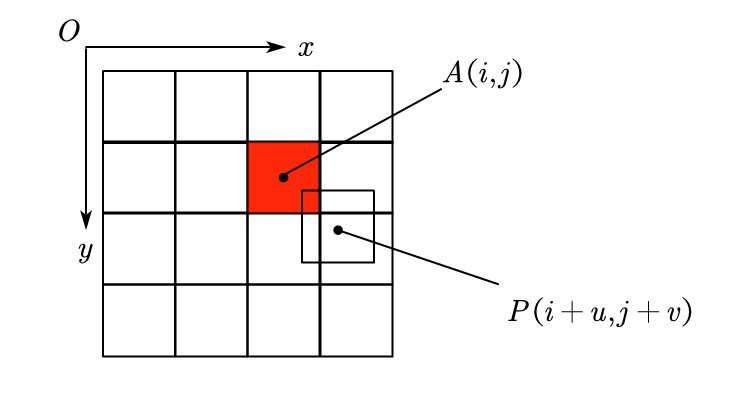图1

1、最近邻插值
算法思路就是将浮点型坐标用int()强制转换为整型，在图2中，浮点型像素点P的坐标被强制转换成整型后，就转为A点，也即用A点单个点的像素代表目标图像矩阵中某个像素值，算法的优点在于速度快，但从图2中就可以看出此算法的误差很大，容易造成图像缩放失真。
2、双线性插值算法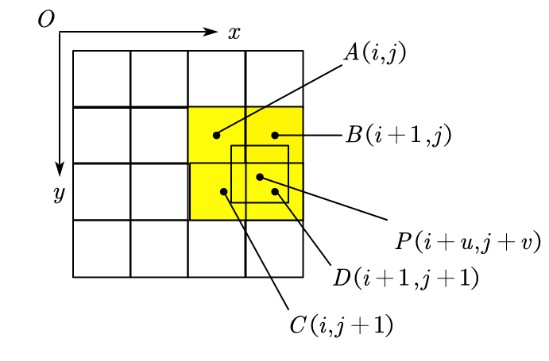图2

算法思路是用浮点型像素点P周围相邻的四个像素，如图2中的A、B、C、D四个点像素的加权平均值来表征P的像素。具体的做法是用横轴、纵轴的距离来表示权重，例如：

△

x

P

,

A

△x_{P,A}

=

u

u

，

△

y

P

,

A

△y_{P,A}

=

v

v

。若用f(M)表示M点的像素值，则P点像素中的A像素分量就为

(

1

−

u

)

(

1

−

v

)

f

(

A

)

(1-u)(1-v)f(A)

显然，u、v越大，P点离A点的距离就越远；那么(1-u)(1-v)就越小，从而A像素f(A)的权重就越小。根据这个思路，可以写出：

f

(

M

)

=

(

1

−

u

)

(

1

−

v

)

f

(

A

)

+

u

(

1

−

v

)

f

(

B

)

+

(

1

−

u

)

v

f

(

C

)

+

u

v

f

(

D

)

f(M)=(1-u)(1-v)f(A)+u(1-v)f(B)+(1-u)vf(C)+uvf(D)

现在要考虑一个目标图像像素坐标得出过程的问题，正如开头所列写的：

s

r

c

X

=

s

r

c

W

i

d

t

h

d

s

t

X

d

s

t

W

i

d

t

h

srcX=srcWidth\frac {dstX}{dstWidth}

s

r

c

Y

=

s

r

c

H

e

i

g

h

t

d

s

t

Y

d

s

t

H

e

i

g

h

t

srcY=srcHeight\frac{dstY}{dstHeight}

如果直接利用这个相似公式，得出的目标图像相对于原图像将不是中心化的，为了说明这一点，假设现在希望将一个5×5的图像缩小为3×3的图像，直接相似关系得出的结果为图3(i)所示，即最右侧和最下侧的像素其实没有参与运算，我们希望得到的图像是如图3(ii)的，这样的放缩才能更多地体现原图像的信息，因此我们需要对放缩公式进行一个补偿修正。图3(i)图3(ii)

考虑将一个m×m的像素矩阵放缩为M×M的像素矩阵，原像素矩阵的中心为(

(

m

−

1

)

2

\frac{(m-1)}{2}

,

(

m

−

1

)

2

\frac{(m-1)}{2}

)，例如一个5×5矩阵，其中心就为(2,2)，若为偶数阶矩阵，其中心可理解为一个虚拟的浮点数像素。将目标像素矩阵的中心(

(

M

−

1

)

2

\frac{(M-1)}{2}

,

(

M

−

1

)

2

\frac{(M-1)}{2}

)代入相似公式，得到(仅列出横坐标，纵坐标同理)：

s

r

c

X

=

(

M

−

1

)

2

m

M

srcX=\frac{(M-1)}{2}\frac{m}{M}

设置一个误差量：

b

i

a

s

=

s

r

c

X

−

(

m

−

1

)

2

bias=srcX-\frac{(m-1)}{2}

化简即得：

b

i

a

s

=

1

2

(

1

−

m

M

)

bias=\frac{1}{2}(1-\frac{m}{M})

因此对相似公式进行修正，得到：

s

r

c

X

=

d

s

t

X

s

r

c

W

i

d

t

h

d

s

t

W

i

d

t

h

−

b

i

a

s

srcX=dstX\frac{srcWidth}{dstWidth}-bias

s

r

c

Y

=

d

s

t

Y

s

r

c

H

e

i

g

h

t

d

s

t

H

e

i

g

h

t

−

b

i

a

s

srcY=dstY\frac{srcHeight}{dstHeight}-bias

这就是中心化公式的由来
二、源代码实现
这里仅贴出双线性插值算法的核心代码段：
for i in range(dstHeight):
for j in range(dstWidth):
srcX = j*(srcWidth/dstWidth)-bias_Width
srcY = i*(srcHeight/dstHeight)-bias_Height
srcX_0 = int(np.floor(srcX))
u = np.float(srcX-srcX_0)
srcX_1 = int(np.ceil(srcX))
if srcX_1>srcWidth-1:             #消除数组越界问题
srcX_1 = srcX_1-1
srcY_0 = int(np.floor(srcY))
v = srcY-srcY_0
srcY_1 = int(np.ceil(srcY))
if srcY_1>srcHeight-1:
srcY_1 = srcY_1-1
dstImgInfo[i][j] =(1-u)*(1-v)*img[srcY_0][srcX_0]+u*(1-v)*img[srcY_0][srcX_1]+(1-u)*v*img[srcY_1][srcX_0]+u*v*img[srcY_1][srcX_1]

代码片部分仅供参考，不进行讲解
三、小结
本篇博客对使用OpenCV的常见API内置算法进行原理性的解析和分享，如有不足还望各位多加指出
展开全文计算机视觉 opencv
• 插值算法最近邻插值、双线性插值 插值算法有很多种，这里列出关联比较密切的三种： 最近邻法(Nearest Interpolation)：计算速度最快，但是效果最差。 双线性插值(Bilinear Interpolation)：双线性插值是用原图像...
插值算法：最近邻插值、双线性插值

文章目录
插值算法：最近邻插值、双线性插值最近邻插值法（nearest_neighbor)线性插值单线性插值法双线性插值

插值算法有很多种，这里列出关联比较密切的三种： 最近邻法(Nearest Interpolation)：计算速度最快，但是效果最差。
双线性插值(Bilinear Interpolation)：双线性插值是用原图像中4(2*2)个点计算新图像中1个点，效果略逊于双三次插值，速度比双三次插值快，属于一种平衡美，在很多框架中属于默认算法。
双三次插值(Bicubic interpolation)：双三次插值是用原图像中16(4*4)个点计算新图像中1个点，效果比较好，但是计算代价过大。

最近邻插值法（nearest_neighbor)
1.原理与应用
最近邻插值法nearest_neighbor是最简单的灰度值插值。也称作零阶插值，就是令变换后像素的灰度值等于距它最近的输入像素的灰度值。最近邻插值法可应用于图像的缩放，因为简单的变换与计算，效果一般不好。举例说明其变换过程：
先假设一个2X2像素的图片采用最近邻插值法需要放大到4X4像素的图片，右边？该为多少。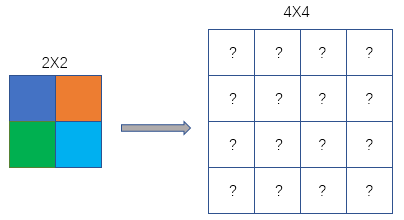2. 公式及计算
最近邻插值法坐标变换计算公式：
srcX=dstX*(srcWidth/dstWidth)
srcY=dstY*(srcHeight/dstHeight)
上式中，dstX与dstY为目标(destination)图像的某个像素的横纵坐标，dstWidth与dstHeight为目标图像的长与宽；srcWidth与srcHeight为原(source)图像的宽度与高度。srcX，srcY为目标图像在该点（dstX，dstY）对应的原图像的坐标。至于公式为啥是这个样，和容易理解，如果是放大图像，(srcWidth/dstWidth)值小于1，同样将dstX同比例映射到原图像的srcX中，如果srcWidth/dstWidth=1，就相当于复制了图片。
图像坐标以左上角为(0,0)坐标。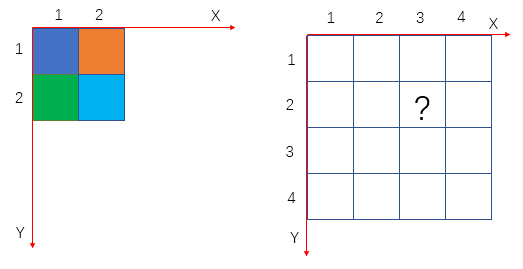右图为经过放大后的目标图像，**？**处的坐标为(3,2)，根据公式计算得到
srcX=3*(2/4)=1.5，srcY=2*(2/4)=1；故？处的像素应该为原图像中的(1.5,1)像素的值，但是像素坐标没有小数，一般采用四舍五入取最邻，所以最终的结果为(2,1)，对应原图像的橙色。其他类比得到放大后的图像：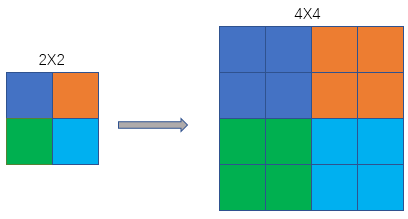线性插值

说双线性之前先说一下单线性，因为在数学上，双线性插值是有两个变量的插值函数的线性插值扩展，其核心思想是在两个方向分别进行一次线性插值。

双线性插值是上采样的一种。在上采样过程中，要恢复图片的大小，提高图片的分辨率，就要用到一些方法，任何可以让图片变成高分辨率的技术都可以称为上采样。

单线性插值法

线性插值法，是指使用连接两个已知量的直线来确定在这两个已知量之间的一个未知量的值的方法。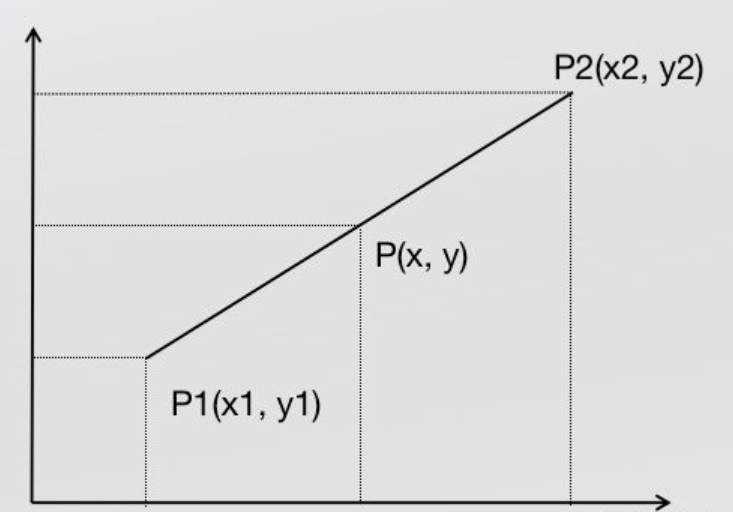根据初中的知识，2点求一条直线公式(这是双线性插值所需要的唯一的基础公式)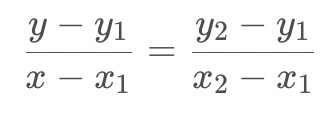经过简单整理成下面的格式：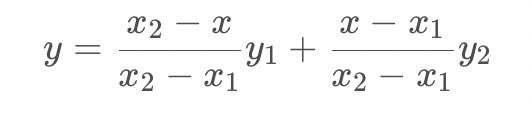这里没有写成经典的AX+B的形式，因为这种形式从权重的角度更好理解。
首先看分子，分子可以看成x与x1和x2的距离作为权重，这也是很好理解的，P点与P1、P2点符合线性变化关系，所以P离P1近就更接近P1，反之则更接近P2。
现在再把公式中的分式看成一个整体，原式可以理解成y1与y2是加权系数，如何理解这个加权，要返回来思考一下，咱们先要明确一下根本的目的：咱们现在不是在求一个公式，而是在图像中根据2个点的像素值求未知点的像素值。这样一个公式是不满足咱们写代码的要求的。
现在根据实际的目的理解，就很好理解这个加权了，y1与y2分别代表原图像中的像素值（x与x1和x2的距离作为权重），上面的公式可以写成如下形式：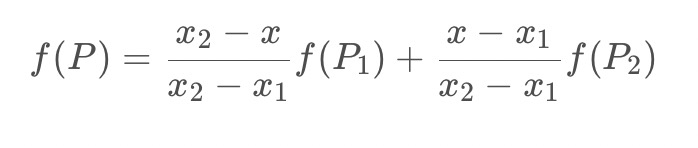双线性插值
根据定义和名字，可以看出就是两个方向的线性插值
假如我们想得到未知函数f在点P= (x,y) 的值，假设我们已知函数f在Q11 = (x1,y1)、Q12 = (x1,y2),Q21 = (x2,y1) 以及Q22 = (x2,y2) 四个点的值。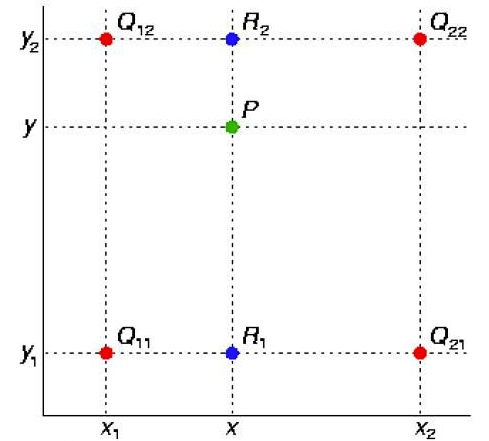首先在x方向进行线性插值，得到R1和R2，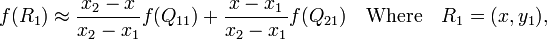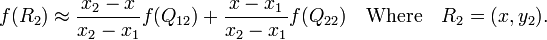然后在y方向进行线性插值，得到P.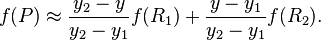整合后的公式如下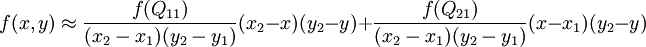2. 图像计算例子
在图像处理的时候，我们先根据
srcX=dstX*(srcWidth/dstWidth)
srcY=dstY*(srcHeight/dstHeight)
来计算目标像素在源图像中的位置（和最近邻插值法一样，但是线性插值的像素是通过公式计算的），这里计算的srcX和srcY一般都是浮点数，
设坐标通过反向变换得到的浮点坐标为 (i+u,j+v) (其中i、j均为浮点坐标的整数部分，u、v为浮点坐标的小数部分，是取值[0,1)区间的浮点数)，则这个像素得值 f(i+u,j+v) 可由原图像中坐标所对应的周围四个像素的值决定，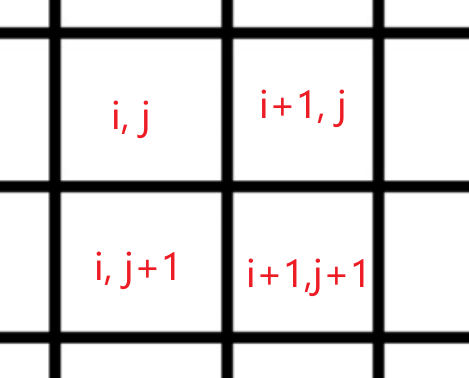计算公式由👆上面整合后的公式替换得到：
f(i+u,j+v) = (1-u)(1-v) f(i,j) + (1-u)v f(i,j+1) + u(1-v) f(i+1,j) + uv f(i+1,j+1)
注：
matlab、openCV对应的resize（）函数的源坐标和目标坐标转换和一般的不一样。
是
SrcX=(dstX+0.5)* (srcWidth/dstWidth) -0.5
SrcY=(dstY+0.5) * (srcHeight/dstHeight)-0.5
python代码
# coding=utf-8
import cv2
import numpy as np
import time

def resize(src, new_size):
dst_w, dst_h = new_size # 目标图像宽高
src_h, src_w = src.shape[:2] # 源图像宽高
if src_h == dst_h and src_w == dst_w:
return src.copy()
scale_x = float(src_w) / dst_w # x缩放比例
scale_y = float(src_h) / dst_h # y缩放比例

# 遍历目标图像，插值
dst = np.zeros((dst_h, dst_w, 3), dtype=np.uint8)
for n in range(3): # 对channel循环
for dst_y in range(dst_h): # 对height循环
for dst_x in range(dst_w): # 对width循环
# 目标在源上的坐标（浮点值）
src_x = (dst_x + 0.5) * scale_x - 0.5
src_y = (dst_y + 0.5) * scale_y - 0.5
# 计算在源图上的（i,j）,和（i+1,j+1）的值
src_x_0 = int(np.floor(src_x))  # floor是下取整
src_y_0 = int(np.floor(src_y))
src_x_1 = min(src_x_0 + 1, src_w - 1)
src_y_1 = min(src_y_0 + 1, src_h - 1)

# 双线性插值
value0 = (src_x_1 - src_x) * src[src_y_0, src_x_0, n] + (src_x - src_x_0) * src[src_y_0, src_x_1, n]
value1 = (src_x_1 - src_x) * src[src_y_1, src_x_0, n] + (src_x - src_x_0) * src[src_y_1, src_x_1, n]
dst[dst_y, dst_x, n] = int((src_y_1 - src_y) * value0 + (src_y - src_y_0) * value1)
return dst

if __name__ == '__main__':
start = time.time()
img_out = cv2.resize(img_in, (600,600))
print('cost %f seconds' % (time.time() - start))

cv2.imshow('src_image', img_in)
cv2.imshow('dst_image', img_out)
cv2.waitKey()

展开全文线性代数 人工智能
• 最近邻插值 最简单的插值方法，顾名思义，选取距离自己最近的一个点的像素值赋给自己。如图所示： 假设P点为带求像素，其在放大区域的坐标为(X,Y)，其x方向和y方向的放大倍数为kx，ky，通过x/kx...计算机视觉
• 这就需要插值算法来进行处理，常见的插值算法最近邻插值、双线性插值和三次样条插值。 1.2 最近邻插值 最近邻插值，是指将目标图像中的点，对应到源图像中后，找到最相邻的整数点，作为插值后的输出。 如上图...计算机视觉
• 插值主要使用利用到，图像放大、缩小、仿射、透射变换上的。 void cvResize( const CvArr* src, CvArr* dst, int interpolation=CV_INTER_LINEAR );...CV_INTER_NN - 最近邻插值, CV_INTER_LINEAR - 双线性插值 (缺
• 插值主要使用利用到，图像放大、缩小、仿射、透射变换上的。 void cvResize( const CvArr* src, CvArr* dst, int interpolation=CV_INTER_...CV_INTER_NN - 最近邻插值, CV_INTER_LINEAR - 双线性插值 (缺省使用) C...
• 最近插值算法原理是在原图像中找到最近临的一个点，然后把这个点的像素值插入到目标图像中，最近插值算法优点是算法简单，易于实现，但是缺点是由于相邻像素点的像素值相同，容易出现色块现象。那么如何在原...
• 对于想自己写算法缩放图像的童鞋比较有用，opencv神马的一般有现成函数的
• 最近邻插值算法原理计算公式效果展示图2.双线性插值算法原理计算公式效果展示图3.映射方法向前映射法向后映射法三.基于opencv的python实现总结 一.简介 在图像处理中，平移变换、旋转变换以及放缩变换是一些基础且...
• 2 Matlab实现最近邻插值、双线性插值、双三次插值3最近邻插值原理简介4双线性插值的原理简介5双三次插值原理简介 1插值是什么？为啥要插值？ 一句话来说就是插值用于图片缩放，为了解决图片缩放中可能出现小数坐标...图片缩放 matlab 双线性插值 双三次插值
• Datawhale学习作者：姚童，Datawhale优秀学习者寄语：本文梳理了最近邻插值法、双线性插值法和三次样条插值法的原理，并以图像缩放为例，对原理进行了C++及Python实现...
• 1.概述 计算机视觉课程要求实现一下图像边缘检测算法，但并没有...Sobel还有一系列延伸的算子，很相似，这些都还是比较简单的，还有一些稍微复杂点的算子，例如Canny，具体关于这些算法原理的介绍推荐去看深入学习Op...边缘检测
• 今天先给出最近邻插值，双线性插值，三次插值。 1.最近邻插值 原始图中影响点数为1 （1）代码 # include&amp;amp;lt;iostream&amp;amp;gt; # include&amp;amp;lt;opencv2/highgui/highgui.hpp&...
• 1.4.1 最近邻插值算法原理   最近邻插值，是指将目标图像中的点，对应到源图像中后，找到最相邻的整数点，作为插值后的输出。 1.4.2 双线性插值   在讲双线性插值之前先看以一下线性插值，线性插值多项式为： f...opencv
• 目录一、插值与图像缩放二、最近邻插值1、原理2、代码实现三、双线性插值1、原理2、代码实现 一、插值与图像缩放   首先举个例子说明插值过程，先看看matlab的插值函数 interp() 吧： x = -2 : 1 : 2; y = -2 : 1 ...
• Python实现双线性插值、最近邻插值、三次内插法 一、最近邻插值法放大图像 最近邻插值法在放大图像时补充的像素是最近邻的像素的值。由于方法简单，所以处理速度很快，但是放大图像画质劣化明显，常常含有锯齿边缘。...python 卷积
• 本文主要讲述最近邻插值(Nearest Neighbor interpolation算法原理以及python实现 基本原理 最简单的图像缩放算法就是最近邻插值。顾名思义，就是将目标图像各点的像素值设为源图像中与其最近的点。算法优点在与...python 机器学习
• 最近邻插值和双线性插值的基本原理 图像的缩放很好理解,就是图像的放大和缩小。传统的绘画工具中,有一种叫做“放大尺”的绘画工具，画家常用它来放大图画。当然，在计算机上，我们不再需要用放大尺去放大或缩小图像...opencv...